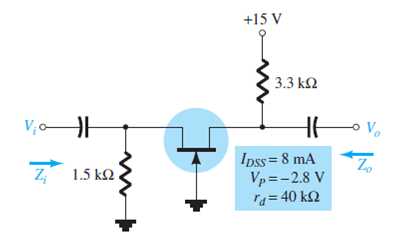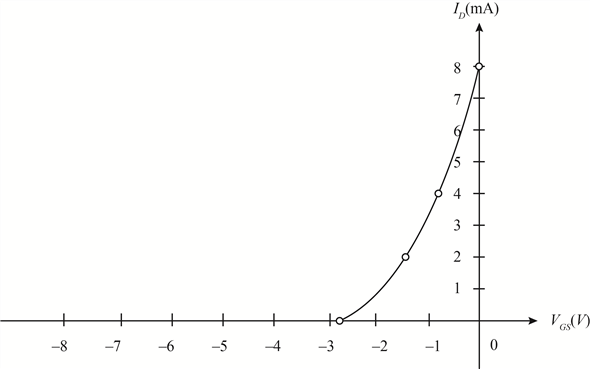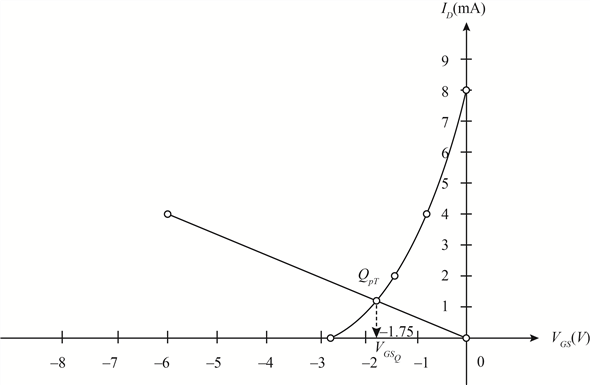Problem

# Determine Zi, Zo, and Vo for the network of Fig. 8.77 if Vi = 4 mV.FIG. 8.77

Determine Zi, Zo, and Vo for the network of Fig. 8.77 if Vi = 4 mV.

FIG. 8.77#### Step-by-Step Solution

Solution 1

Refer to Figure $$8.77$$ in the textbook.

Expression for drain current $$\left(I_{D}\right)$$ is,

$$I_{D}=I_{D S S}\left(1-\frac{V_{G S}}{V_{P}}\right)^{2}$$

Here,

Drain saturation current $$\left(I_{D S S}\right)$$ is $$8 \mathrm{~mA}$$,

Pinch-off voltage $$\left(V_{P}\right)$$ is $$-2.8 \mathrm{~V}$$,

Gate-source voltage is $$V_{G S}$$

Calculate the drain current $$\left(I_{D}\right)$$ at $$V_{G S}=0$$.

\begin{aligned} I_{D} &=(8 \mathrm{~mA})\left[1-\frac{0}{(-2.8)}\right]^{2} \\ &=8 \mathrm{~mA} \end{aligned}

Calculate the drain current $$\left(I_{D}\right)$$ at $$V_{G S}=V_{P}$$.

$$V_{G S}=V_{P}$$

\begin{aligned} &=-2.8 \mathrm{~V} \\ I_{D} &=(8 \mathrm{~mA})\left[1-\frac{(-2.8)}{(-2.8)}\right]^{2} \\ &=0 \mathrm{~mA} \end{aligned}

Calculate drain current $$\left(I_{D}\right)$$ at $$V_{G S}=\frac{V_{p}}{2}$$.

\begin{aligned} V_{G S} &=\frac{V_{p}}{2} \\ &=-1.4 \mathrm{~V} \\ I_{D} &=(8 \mathrm{~mA})\left[1-\frac{\left(\frac{-2.8}{2}\right)}{(-2.8)}\right]^{2} \\ &=2 \mathrm{~mA} \end{aligned}

Calculate drain current $$\left(I_{D}\right)$$ at $$V_{G S}=0.3 V_{P} .$$

$$V_{G S}=0.3 V_{P}$$

\begin{aligned} &=-0.84 \mathrm{~V} \\ I_{D} &=(8 \mathrm{~mA})\left[1-\frac{(0.3)(-2.8)}{(-2.8)}\right]^{2} \\ &=3.92 \mathrm{~mA} \\ & \cong 4 \mathrm{~mA} \end{aligned}

The transfer curve for the device is shown in Figure 1:Figure 1: Required schematic

To sketch load line locates two coordinates in figure 1 and draw load line as follows:

Calculate gate source voltage $$\left(V_{G s_{Q}}\right)$$

$$V_{G S}=-I_{D} R_{s} \ldots \ldots$$ (1)

Here,

Source resistance $$\left(R_{S}\right)$$ is $$1.5 \mathrm{k} \Omega$$

Let $$I_{D}=0$$

Substitute $$1.5 \mathrm{k} \Omega$$ for $$R_{S}$$ and 0 for $$I_{D}$$ in equation (1).

$$V_{G S}=-(0)(1.5 \mathrm{k} \Omega)$$

$$=0 \mathrm{~V}$$

Therefore the gate source voltage corresponding to $$0 \mathrm{~mA}$$ drain current is $$0 \mathrm{~V}$$.

Again solve equation (1) for $$I_{D}=4 \mathrm{~A}$$.

\begin{aligned} V_{G S} &=-(4 \mathrm{~mA})(1.5 \mathrm{k} \Omega) \\ &=-6 \mathrm{~V} \end{aligned}

Therefore the gate source voltage corresponding to $$4 \mathrm{~mA}$$ drain current is $$-6 \mathrm{~V}$$

The point of intersection of the load line and a transverse curve is defined as $$Q$$ -point.

Here, the load line cuts the transverse curve at $$V_{G S_{Q}} \cong-1.75 \mathrm{~V}$$.

The required graph is shown in Figure 2:Figure 2 Required schematic.

Calculate transconductance $$\left(g_{m o}\right):$$

$$g_{m o}=\frac{2 I_{D S S}}{\left|V_{P}\right|}$$

Here,

Drain saturation current $$\left(I_{D S s}\right)$$ is $$8 \mathrm{~mA}$$

Pinch-off voltage $$\left(V_{P}\right)$$ is $$-2.8 \mathrm{~V}$$

Substitute $$-2.8 \mathrm{~V}$$ for $$V_{P}$$ and $$8 \mathrm{~mA}$$ for $$I_{D s s}$$ in the expression of transconductance $$\left(g_{m o}\right)$$

\begin{aligned} g_{m o} &=\frac{2(8 \mathrm{~mA})}{|-2.8 \mathrm{~V}|} \\ &=\frac{16 \mathrm{~mA}}{2.8 \mathrm{~V}} \\ &=5.714 \mathrm{mS} \end{aligned}

Calculate transconductance $$\left(g_{m}\right)$$

$$g_{m}=g_{m o}\left(1-\frac{V_{G S_{\rho}}}{V_{P}}\right)$$

Substitute $$-2.8 \mathrm{~V}$$ for $$V_{P}$$ and $$-1.75 \mathrm{~V}$$ for $$V_{G S_{Q}}$$ and $$5.714 \mathrm{mS}$$ for $$g_{m o}$$ in the expression of transconductance $$\left(g_{m}\right) .$$

\begin{aligned} g_{m} &=(5.714 \mathrm{mS})\left[1-\frac{(-1.75 \mathrm{~V})}{(-2.8 \mathrm{~V})}\right] \\ &=(5.714 \mathrm{mS})(1-0.625) \\ &=(5.714 \mathrm{mS})(0.375) \\ &=2.14 \mathrm{mS} \end{aligned}

Check approximation condition

$$r_{d} \geq 10 R_{D}$$

Here,

Small signal input impedance $$r_{d}$$ is $$40 \mathrm{k} \Omega$$

Drain resistance $$R_{D}$$ is $$3.3 \mathrm{k} \Omega$$

Substitute $$3.3 \mathrm{k} \Omega$$ for $$R_{D}$$ and $$40 \mathrm{k} \Omega$$ for $$r_{d}$$ in approximation condition

$$\begin{array}{l} 40 \mathrm{k} \Omega \geq 10(3.3 \mathrm{k} \Omega) \\ 40 \mathrm{k} \Omega \geq 33 \mathrm{k} \Omega \end{array}$$

Calculate input impedance $$\left(Z_{i}\right)$$

\begin{aligned} Z_{i}=& R_{S} \| \frac{1}{g_{m}} \\ =& \frac{\left(R_{S}\right)\left(\frac{1}{g_{m}}\right)}{R_{S}+\frac{1}{g_{m}}} \end{aligned}

Substitute $$1.5 \mathrm{k} \Omega$$ for $$R_{S}$$ and $$2.14 \mathrm{mS}$$ for $$g_{m}$$ in the expression of input impedance $$\left(Z_{i}\right)$$

\begin{aligned} Z_{i} &=\frac{(1.5 \mathrm{k} \Omega)(467 \Omega)}{1.5 \mathrm{k} \Omega+(467 \Omega)} \\ &=356.3 \Omega \end{aligned}

Hence, the input impedance $$\left(Z_{i}\right)$$ is $$356.3 \Omega$$.

As $$r_{d} \geq 10 R_{D}$$, therefore output impedance $$\left(Z_{o}\right)$$ is equal to drain resistance $$\left(R_{D}\right)$$.

$$Z_{o}=R_{D}$$

Substitute $$3.3 \mathrm{k} \Omega$$ for $$R_{D}$$ in the expression of output impedance $$\left(Z_{o}\right)$$.

$$Z_{o}=3.3 \mathrm{k} \Omega$$

Hence, the output impedance is $$3.3 \mathrm{k} \Omega$$.

As $$r_{d} \geq 10 R_{D}$$, therefore calculate voltage gain $$\left(A_{v}\right)$$

$$A_{v}=g_{m} R_{D}$$

Substitute $$3.3 \mathrm{k} \Omega$$ for $$R_{D}$$ and $$2.14 \mathrm{mS}$$ for $$g_{m}$$ in the expression of voltage gain $$\left(A_{v}\right)$$.

\begin{aligned} A_{v} &=(2.14 \mathrm{mS})(3.3 \mathrm{k} \Omega) \\ &=7.062 \end{aligned}

Hence, the voltage gain $$\left(A_{v}\right)$$ is $$7.062$$.

Calculate output voltage $$\left(V_{o}\right)$$

$$V_{o}=A_{v} V_{i}$$

Here,

Input voltage $$\left(V_{i}\right)$$ is $$4 \mathrm{~V}$$.

Substitute $$4 \mathrm{~V}$$ for $$V_{i}$$ and $$7.062 \mathrm{~V}$$ for $$A_{v}$$ in the expression of $$\left(V_{o}\right)$$

$$V_{o}=(7.06)(4 \mathrm{~V})$$

$$=28.248 \mathrm{~V}$$

Hence, the output voltage $$\left(V_{o}\right)$$ is $$28.248 \mathrm{~V}$$.